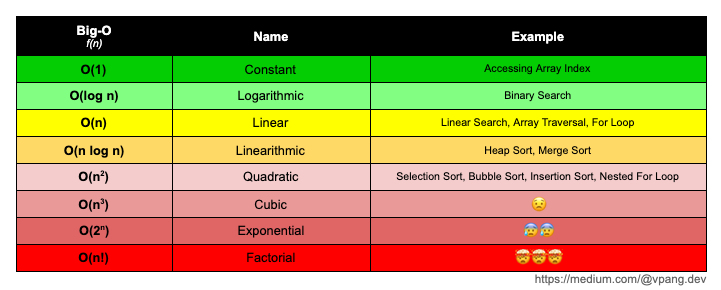# Asymptotic Runtime Complexity — Analyzing Time and SpaceMy definition of Big-O Notation.
`(a+b)/a = a/b = φ`

# A Simple Guide to Big-O NotationIf your algorithm is O(n²) or greater, it’s time to reconsider and start refactoring.

# Calculating the Big-O

`// O(1)function(str){   console.log(str)};// O(n)function printArray(arr){   for(let i = 0; i < arr.length; i++){      ...   }};// O(n log n)function sumOfTwoNum (arr, sum){   const sortedArr = arr.sort(( a , b ) => a - b);   let left = 0;   let right = arr.length - 1;   while (left !== right){       ...   }};// O(n^2)function iterateArray(arr){   for(let i = 0; i < arr.length; i++){      for(let j = 0; j < arr.length; j++){         ...      }   }};// O(2^n)function fib(num){   if(num < 2){      return num   }   return fib(num - 1) + fib(num - 2)};`
`const reverseString = (str) => {    let splitStr = str.split('')                     //O(n)    let reverseStr = splitStr.reverse().join('')     //O(n)    return reverseStr}`
`const iterateArray = (arr) => {   for(let i = 0; i < arr.length; i++){      //O(n)      ...   }      for(let i = 0; i < arr.length; i++){      //O(n^2) = O(n) * O(n)      for(let j = 0; j < arr.length; j++){         ...      }   }};`

## More from Vincent Pang

https://www.linkedin.com/in/vincentpangu